# SGI-STL源码剖析之RB-tree

### 平衡二叉搜索树

AVL tree是一个“加上了额外平衡条件”的二叉搜索树。其平衡条件的建立是为了确保整棵树的深度为O（logN） 。直观上的最佳平衡条件是每个节点的左右子树有着相同的高度，但这未免太过严苛。 AVL tree 于是退而求其次，其平衡条件为：任何节点的左右子树高度相差最多 1 。这是一个较弱的条件，但仍能够保证“对数深度 (logarithmic depth) ”平衡状态。

1．插人点位于 X 的左子节点的左子树——左左。
2．插入点位于 X 的左子节点的右子树——左右。
3．插入点位于 X 的右子节点的左子树——右左。
4．插入点位于 X 的右子节点的右子树——右右。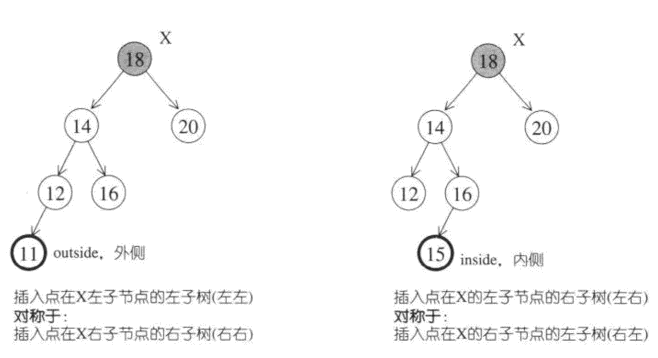### RB tree( 红黑树 )

RB— tree 不仅是一个二叉搜索树，而且必须满足以下规则 ( 即平衡条件 ) ：
1． 每个节点不是红色就是黑色
2 ．根节点为黑色。
3 ．如果节点为红，其子节点必须为黑。
4．任一节点至 NULL( 树尾端 ) 的任何路径，所含之黑节点数必须相同。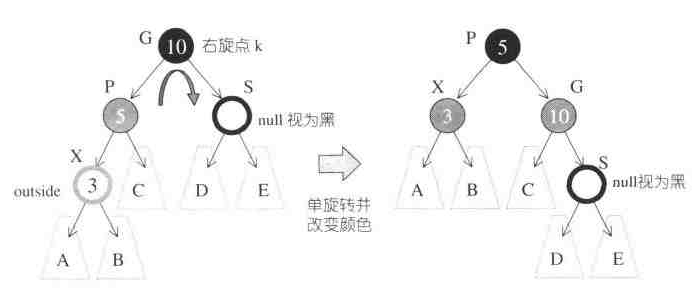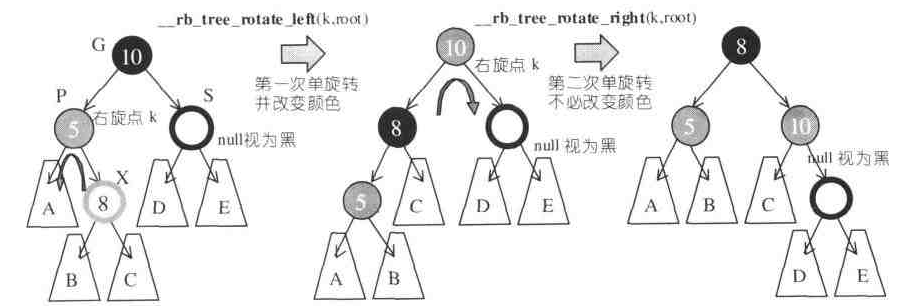《 STL 源码剖析》原文中的状况 3,4 **
**状况 3：
S为红且 X 为外侧插入。对此情况，先对 P 和 G 做一次单旋转，并改变 X 的颜色：此时如果 GG 为黑，一切搞定。如下图，但如果 GG 为红，则问题就比较大些，见状况 4 ．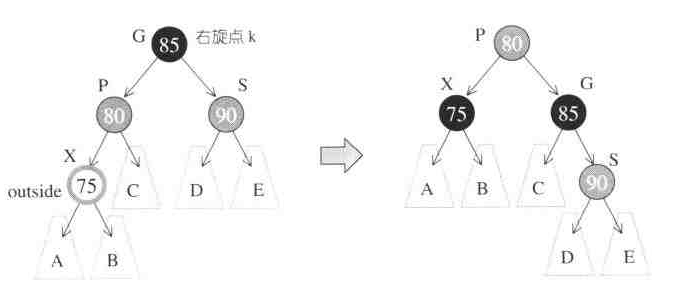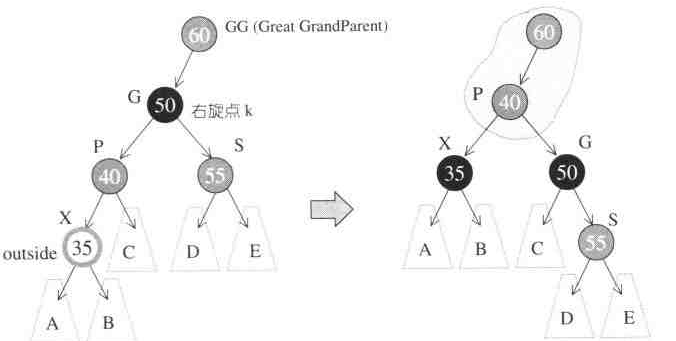**状况 3：
S 为红且 X 为外侧插入，或者为内侧插入。对此情况，改变 P,G,S 颜色，此时如果 GG 为黑，一切搞定。如果 GG 为红，见状况 4 。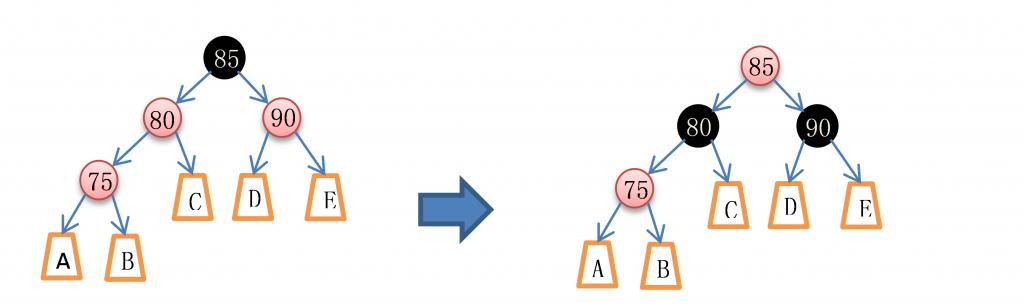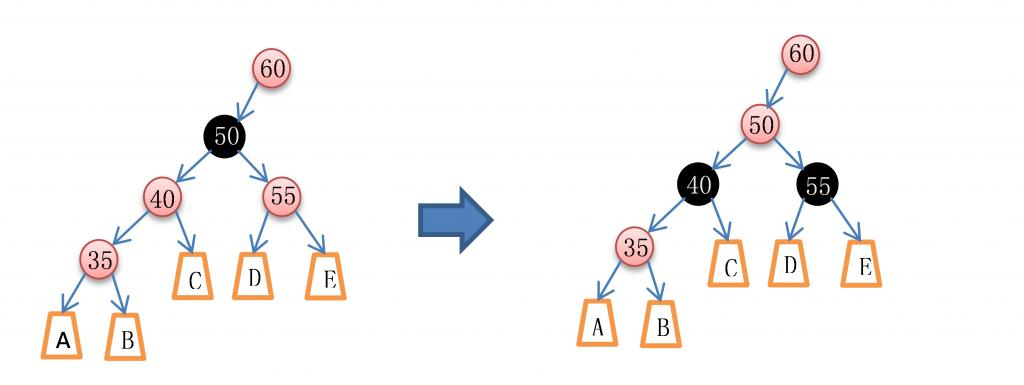#### RB-tree 迭代器

``````void increment ()
{
if (node—>right!=0)       // 如果有右子节点。 状况 <A>
{
node=node—>right ；    // 就向右走
while(node—>left! ； )// 然后一直往左子树走到底
node=node—>left ； // 即是解答
}else {                       // 没有右子节点。 状况 <B>
base_ptr y=node—>parent ； // 找出父节点
while (node==y—>right){   // 如果现行节点本身是个右子节点
node=y ；            // 就一直上溯，直到不为右子节点为止
y=y->parent ；
}
if (node—>right!=y)        // 若此时的右子节点不等于此时的父节点
node=y ；              // 状况 <C> 此时的父节点即为解答
// 否则此时的 node 为解答 状况 <D>
}
// 注意，以上判断 “ 若此时的右子节点不等于此时的父节点 ” ，是为了应付一种
// 特殊情况：我们欲寻找根节点的下一节点，而恰巧根节点无右子节点
// 当然，以上特殊做法必须配合 RB—tree 根节点与特殊之 header 之间的
// 特殊关系
}

void decrement ()
{
if (node—>color==_rbtree_red&&          // 如果是红节点，且
node—>parent—>parent==node)      // 父节点的父节点等于自己，
node=node—>right ；               // 状况 <A> 右子节点即为解答
// 以上情况发生于 node 为 header 时亦即 node 为 end() 时 )
// 注意， header 之右子节点即 most right ，指向整棵树的 max 节点
else if (node—>left!=0){          // 如果有左子节点。 状况 <B>
base_ptry=node—>left ；     // 令 y 指向左子节点
while (y—>right!=0)     // 当 y 有右子节点时
y=y—>right ；      // 一直往右子节点走到底
node=y ；         // 最后即为答案
} else {     // 既非根节点，亦无左子节点
base_ptry=node—>parent ；          // 找出父节点 状况 <C>
while (node 二二 y—>left){       // 当现行节点身为左子节点
node=y ；                 // 一直交替往上走，直到现行节点
y=y—>parent ；            // 不为左子节点
}
node=y ； // 此时之父节点即为答案
}
}``````

increment() 和 decrement() 两函数中，较为令人费解的是前者的状况< D >和后者的状况< A >，他们分别发生在下图所展示的状态下：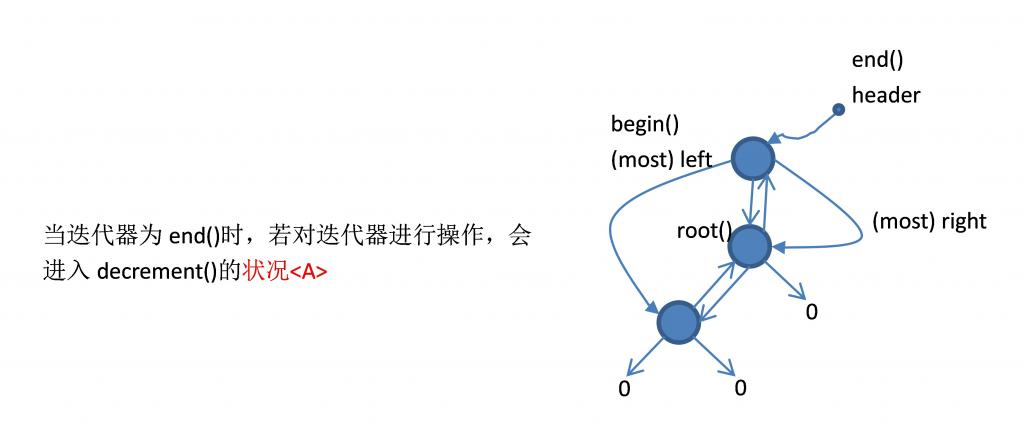#### RB-tree 的数据结构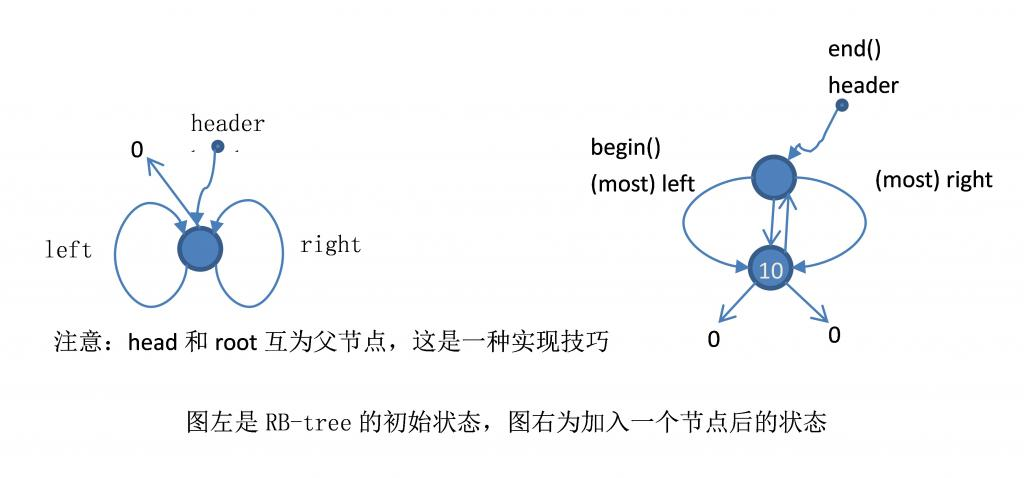#### 调整 RB-tree

``````// 重新令树形平衡（改变颜色及旋转树形）
// 参数一为新增节点，参数二为 root
inline void
__rb_tree_rebalance (__rb_tree_node_base* x , __rb_tree_node_base*& root )
{
x->color = __rb_tree_red;         // 新节点必为红
while (x != root && x->parent->color == __rb_tree_red) { // 父节点为红
// 父节点 P 为祖父节点 G 的左子节点 , 以下将进行已分析的四种状况。
if (x->parent == x->parent->parent->left) { // 父节点为祖父节点之左子节点
__rb_tree_node_base* y = x->parent->parent->right;     // 令 y 为伯父节点
// 状态 3 ：父节点为祖父节点之左节点，伯父节点存在且为红，下面的操作仅仅改变颜色，
// 可以证明《 STL 源码剖析》状况 3 有错
if (y && y->color == __rb_tree_red) {     // 伯父节点存在，且为红
x->parent->color = __rb_tree_black;      // 更改父节点为黑
y->color = __rb_tree_black;               // 更改伯父节点为黑
x->parent->parent->color = __rb_tree_red;    // 更改祖父节点为红
// 状态 4 ：准备继续向上检查，即为状态 4 做检查准备工作 , 看到外层的 while 循环了吗？
x = x->parent->parent;
}
else {     // 无伯父节点，或伯父节点为黑
// 状态 2 ：无伯父节点，或伯父节点为黑， X 为父节点 P 的右子节点，即内侧插入，所以需要右旋转，
// 然后继续向下完成左旋转
if (x == x->parent->right) { // 如果新节点为父节点之右子节点
x = x->parent;
__rb_tree_rotate_left (x, root); // 第一参数为左旋点
}

// 状态 1 ：无伯父节点，或伯父节点为黑， X 为父节点 P 的左子节点，即外侧插入，仅仅需要左旋转。
// 状态 2 的左旋转同样在此完成
x->parent->color = __rb_tree_black;   // 改变颜色
x->parent->parent->color = __rb_tree_red;
__rb_tree_rotate_right (x->parent->parent, root); // 第一参数为右旋点
}
}
// 父节点 P 为祖父节点 G 的左子节点 , 类似上述四种状况。
else {   // 父节点为祖父节点之右子节点
__rb_tree_node_base* y = x->parent->parent->left; // 令 y 为伯父节点
if (y && y->color == __rb_tree_red) {      // 有伯父节点，且为红
x->parent->color = __rb_tree_black;       // 更改父节点为黑
y->color = __rb_tree_black;              // 更改伯父节点为黑
x->parent->parent->color = __rb_tree_red;    // 更改祖父节点为红
x = x->parent->parent;    // 准备继续往上层检查 ...
}
else {     // 无伯父节点，或伯父节点为黑
if (x == x->parent->left) {   // 如果新节点为父节点之左子节点
x = x->parent;
__rb_tree_rotate_right (x, root);    // 第一参数为右旋点
}
x->parent->color = __rb_tree_black;   // 改变颜色
x->parent->parent->color = __rb_tree_red;
__rb_tree_rotate_left (x->parent->parent, root); // 第一参数为左旋点
}
}
}     // while 结束
root->color = __rb_tree_black;    // 修正根节点，根节点永远为黑
}

// 新节点必为红节点。如果安插处之父节点亦为红节点，就违反红黑树规则，此时必须
// 做树形旋转（及颜色改变，在程序它处）。
inline void
__rb_tree_rotate_left (__rb_tree_node_base* x , __rb_tree_node_base*& root )
{
// x 为旋转点
__rb_tree_node_base* y = x->right;    // 令 y 为旋转点的右子节点
x->right = y->left;
if (y->left !=0)
y->left->parent = x;         // 别忘了回马枪设定父节点
y->parent = x->parent;
// 令 y 完全顶替 x 的地位（必须将 x 对其父节点的关系完全接收过来）
if (x == root)                    // x 为根节点
root = y;
else if (x == x->parent->left)    // x 为其父节点的左子节点
x->parent->left = y;
else                          // x 为其父节点的右子节点
x->parent->right = y;

y->left = x;
x->parent = y;
}

// 新节点必为红节点。如果安插处之父节点亦为红节点，就违反红黑树规则，此时必须
// 做树形旋转（及颜色改变，在程序它处）。
inline void
__rb_tree_rotate_right (__rb_tree_node_base* x , __rb_tree_node_base*& root )
{
// x 为旋转点
__rb_tree_node_base* y = x->left;     // y 为旋转点的左子节点
x->left = y->right;
if (y->right != 0)
y->right->parent = x;   // 别忘了回马枪设定父节点
y->parent = x->parent;

// 令 y 完全顶替 x 的地位（必须将 x 对其父节点的关系完全接收过来）
if (x == root)                    // x 为根节点
root = y;
else if (x == x->parent->right)   // x 为其父节点的右子节点
x->parent->right = y;
else                          // x 为其父节点的左子节点
x->parent->left = y;

y->right = x;
x->parent = y;
}``````